18 篇文章 9 订阅

# 1. 概述

1）从目标图像（target image）通过编解码网络预测深度信息；
2）从源图像（source images）和目标图像组成的图像对中去估计出该图像对之间的变换关系（由旋转和平移组成的6维度变换状态）；

1）使用最小重构误差损失作为最后的损失函数，增加对于视频序列中存在遮挡情况的鲁棒性，以及解决平均重构误差损失带来的模糊问题；
2）使用多尺度预测（统一resize在训练图分辨率上）并计算损失，不仅避免了陷入局部最优，而且使得深度的监督输入图片分辨率下更有指向性，从而减少视觉伪影的产生；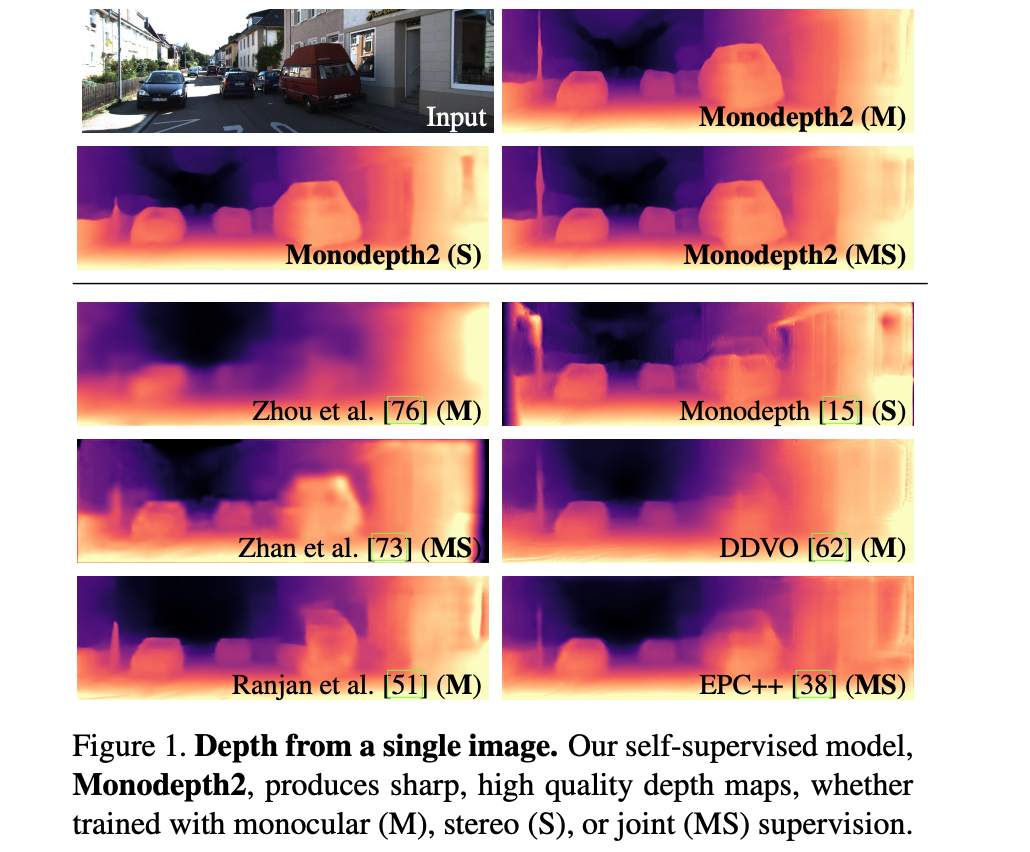# 2. 方法设计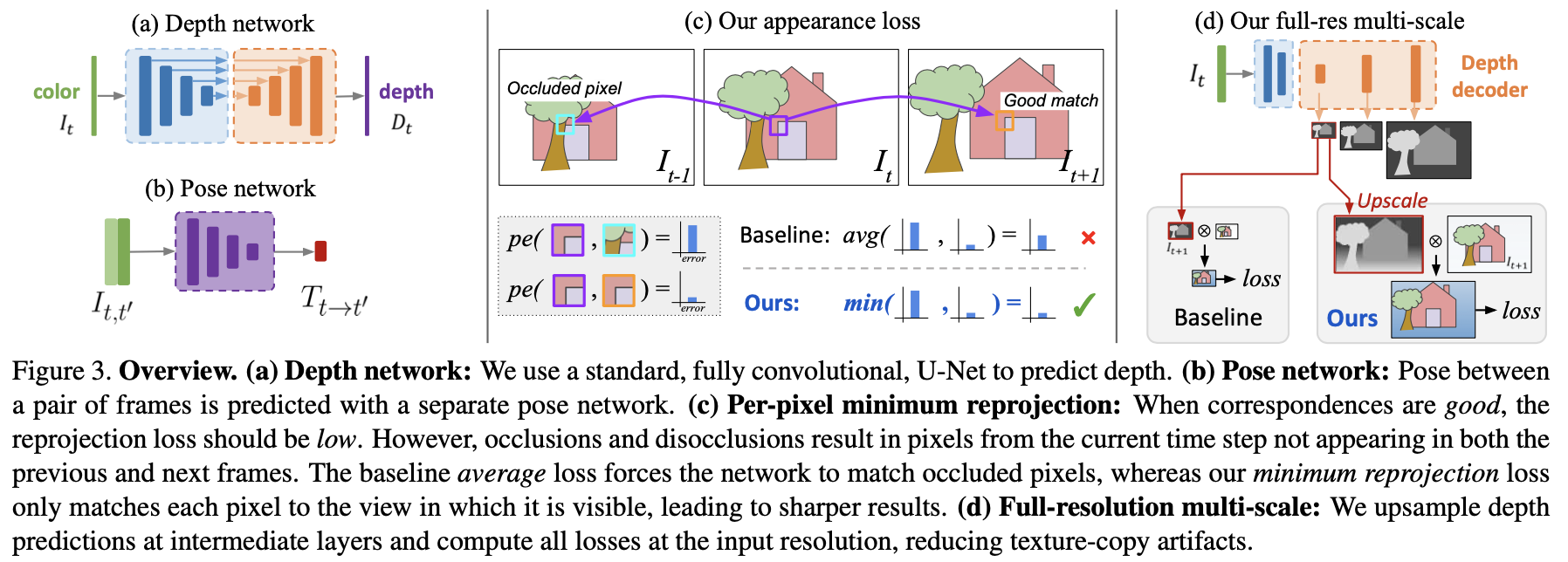## 2.2 自监督训练

L p = ∑ t ‘ p e ( I t , I t ‘ → t ) L_p=\sum_{t^{‘}}pe(I_t,I_{t^{‘}\rightarrow t})

I t ‘ → t = I t ‘ ⟨ p r o j ( D t , T t → t ‘ , K ) ⟩ I_{t^{‘}\rightarrow t}=I_{t^{‘}}\langle{proj(D_t,T_{t\rightarrow t^{‘}},K)}\rangle

p e ( I a , I b ) = α 2 ( 1 − S S I M ( I a , I b ) ) + ( 1 − α ) ∣ ∣ I a − I b ∣ ∣ 1 pe(I_a,I_b)=\frac{\alpha}{2}(1-SSIM(I_a,I_b))+(1-\alpha)||I_a-I_b||_1

L s = ∣ ∂ x d t ∗ ∣ e − ∣ ∂ x I t ∣ + ∣ ∂ y d t ∗ ∣ e ∂ y I t L_s=|\partial_xd_t^{*}|e^{-|\partial_xI_t|}+|\partial_yd_t^{*}|e^{\partial_yI_t}

## 2.2 自监督训练中的策略

• 1）由于如相机运动而导致一些像素移出了图像的边界；
• 2）由于视频中目标移动导致视频中的目标存在遮挡；

L p = min ⁡ t ‘ p e ( I t , I t ‘ → t ) L_p=\min_{t^{‘}}pe(I_t,I_{t^{‘}\rightarrow t})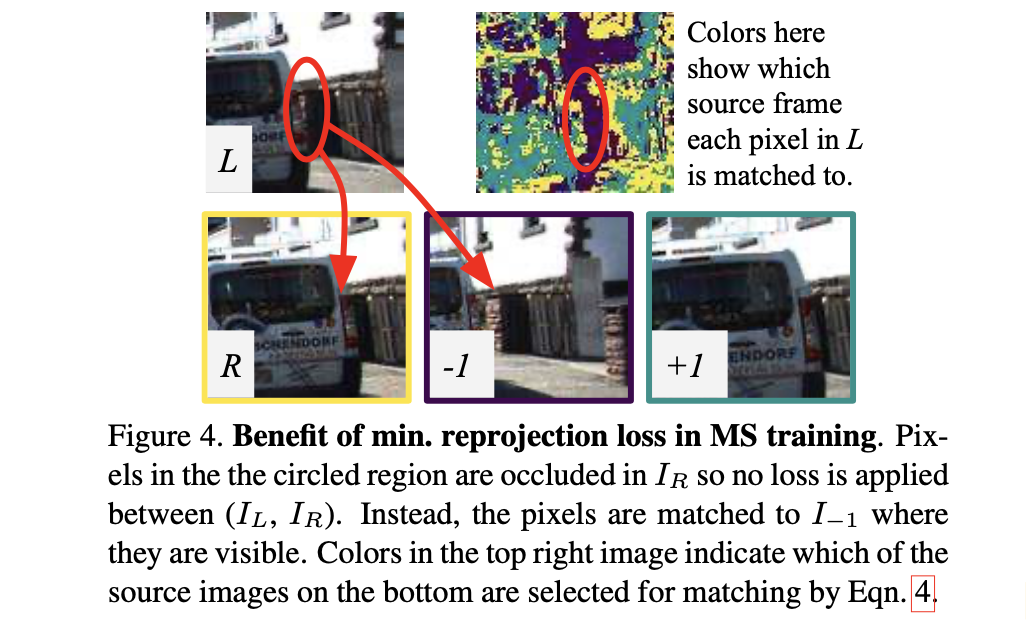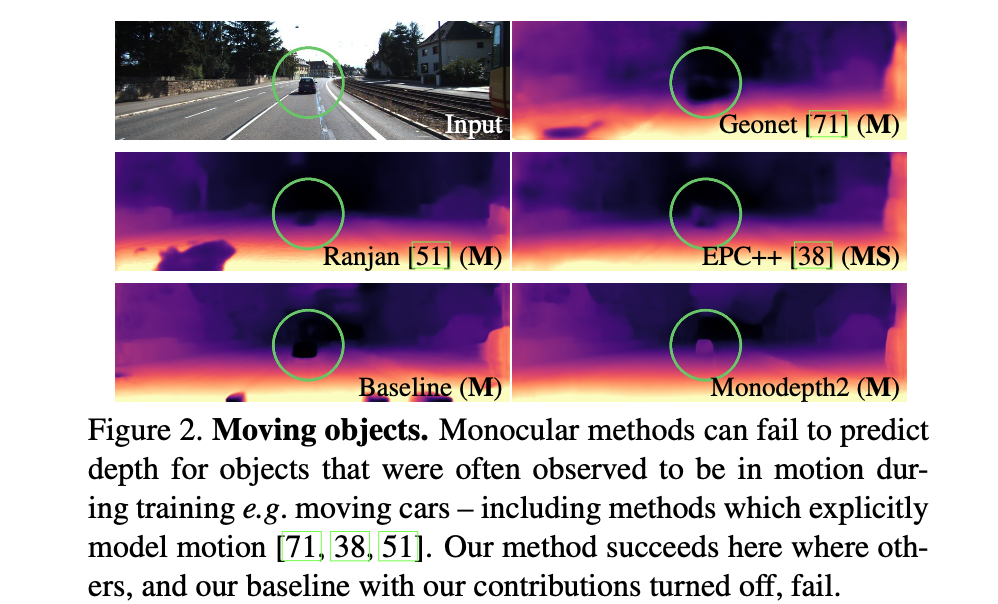μ = min ⁡ t ’ p e ( I t , I t ’ → t ) < min ⁡ t ’ p e ( I t , I t ’ ) \mu=\min_{t^{’}}pe(I_t,I_{t^{’}\rightarrow t})\lt\min_{t^{’}}pe(I_t,I_{t^{’}})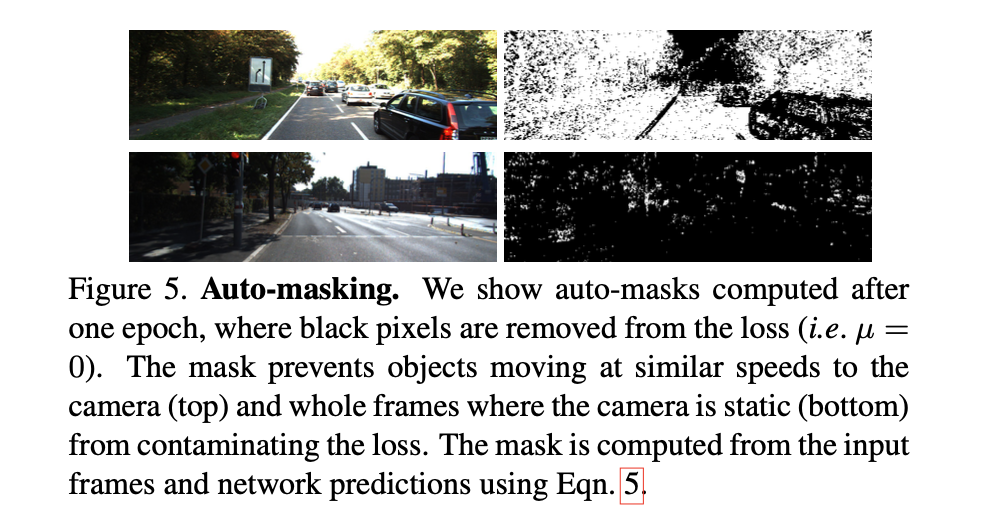## 2.3 损失函数

L = μ L p + λ L s L=\mu L_p+\lambda L_s

# 3. 实验结果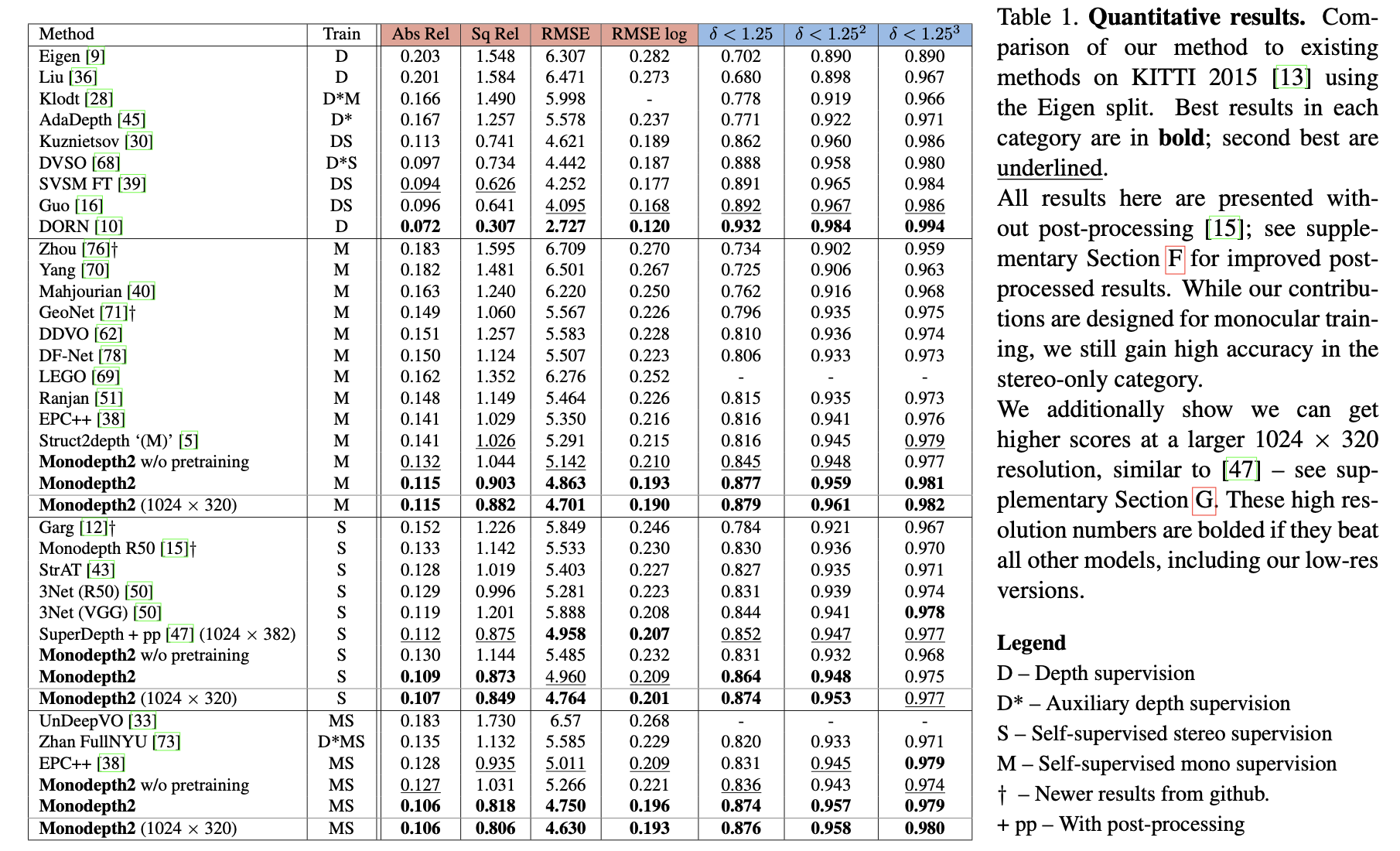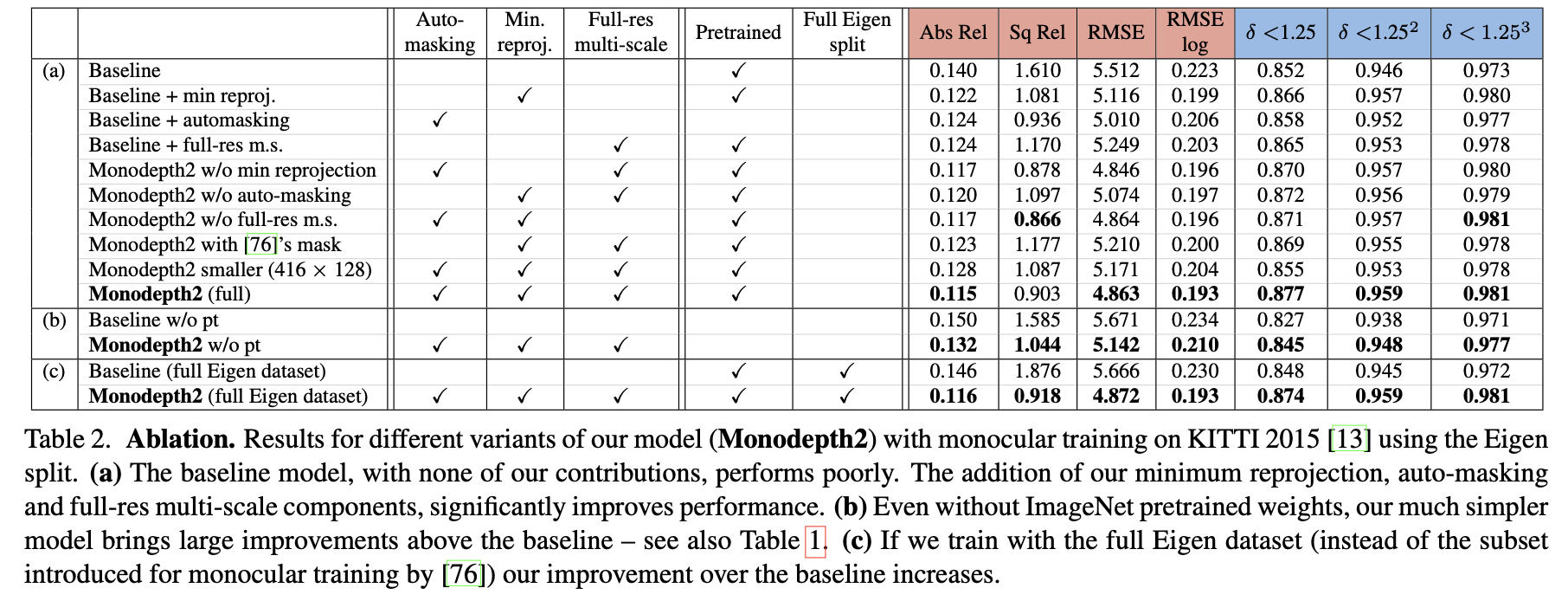12-11179407-305816
08-02182
12-1978
05-17349
04-122819
10-163381
06-16115
06-13550
08-06390
12-091281
06-14393
04-203973
04-0968
04-011348点击重新获取扫码支付余额充值